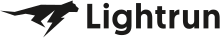• Dev Observability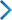• Product• Pricing
• Docs
• Resources• Blog
• Company• Debug Wordle# Stuck on an issue?

Lightrun Answers was designed to reduce the constant googling that comes with debugging 3rd party libraries. It collects links to all the places you might be looking at while hunting down a tough bug.

And, if you’re still stuck at the end, we’re happy to hop on a call to see how we can help out.

See original GitHub issue

### Issue Description

Consider the following function. It generates and solves a model structurally somewhat similar to a practical problem I am solving. (I tried to simplify it as much as possible)

``````def experiment_mip(n):
start = time.time()

model = mip.Model(sense=mip.MAXIMIZE, solver_name=mip.CBC)
obj = []
for i in range(n):
a = random.random()  - 0.5  # Just a dummy value for illustration
obj.append(var * a)
constraint = mip.xsum(obj) <= 1.0
model.objective = mip.xsum(obj)

construction_time = time.time() - start
start = time.time()

model.optimize()

solution_time = time.time() - start
return {"Model construction time": construction_time, "Solution time": solution_time}
``````

Now, try running this for example for `n=4000`. The solution is much faster than the construction of the model. My measurements suggest that the solution time is asymptotically `O(n^2)` complexity but the construction is more than `O(n^3)`. The profiler shows that that most time is spent in the `LinExpr.add_var`, `LinExpr.add_expr` and `LinExpr.add_term` functions. I think this is not acceptable and it should never take more time to construct than to solve a nontrivial problem.

I am not sure what the solution could be.

### Issue Analytics

• State: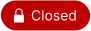• Created 2 years ago
• Reactions:2

####Top GitHub Comments

1reaction
tuliotoffolocommented, Sep 24, 2021

I’ve just included a test considering 5 variations of the code @jachymb and @christian2022 posted here. The test example is available in this link and here are the ‘construction’ runtimes I got with n=10000:

``````using_addvar: 9.426069974899292
using_inplace_op: 9.878501176834106
using_dict: 11.112802028656006
using_lists: 14.583881855010986
recreating_linexpr: 74.6143479347229
``````

@rschwarz: you can now create a LinExpr from a dictionary or two lists (variables and coeffs).

1reaction
christian2022commented, May 25, 2021

With these minor changes (see `###`), on my laptop construction time is 1/3 of solution time for `n = 4000`

``````def experiment_mip(n):
start = time.time()

model = mip.Model(sense=mip.MAXIMIZE, solver_name=mip.CBC)
obj = LinExpr() ### do not build array of LinExpr but directly append to LinExpr
for i in range(n):
a = random.random()  - 0.5  # Just a dummy value for illustration
obj.add_var(var, a) ### do not create intermediate LinExpr
constraint = obj <= 1.0 ### no need to mip.xsum as obj is already the sum
model.objective = obj

construction_time = time.time() - start
start = time.time()

model.optimize()

solution_time = time.time() - start
return {"Model construction time": construction_time, "Solution time": solution_time}
``````

####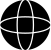Top Results From Across the Web

I need help with LinExpr - Gurobi Support Portal
I am trying to code a simple objective function which works fine. I read that quicksum() isn't the best way to model large...
how to fix 'gurobipy.LinExpr' object is not iterable
I am quite new to programming, and don't really understand what the error is. It comes up as 'gurobipy.LinExpr' object is not iterable...
Var' object is not iterable, TypeError: 'gurobipy.LinExpr' object ...
Hi,. There are a few issues in your code. First of all the model is not convex and so Gurobi will not solve...
gurobi::LinExpr - Rust - Docs.rs
API documentation for the Rust `LinExpr` struct in crate `gurobi`.
Python Examples of gurobipy.LinExpr - ProgramCreek.com
def fit(array, convex=1): """Fit a smooth line to the given time-series data""" N = len(array) m = gurobipy.Model() fv = m.addVars(N) if convex...

####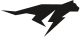Troubleshoot Live Code

Lightrun enables developers to add logs, metrics and snapshots to live code - no restarts or redeploys required.
Start Free

####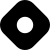Top Related Hashnode Post